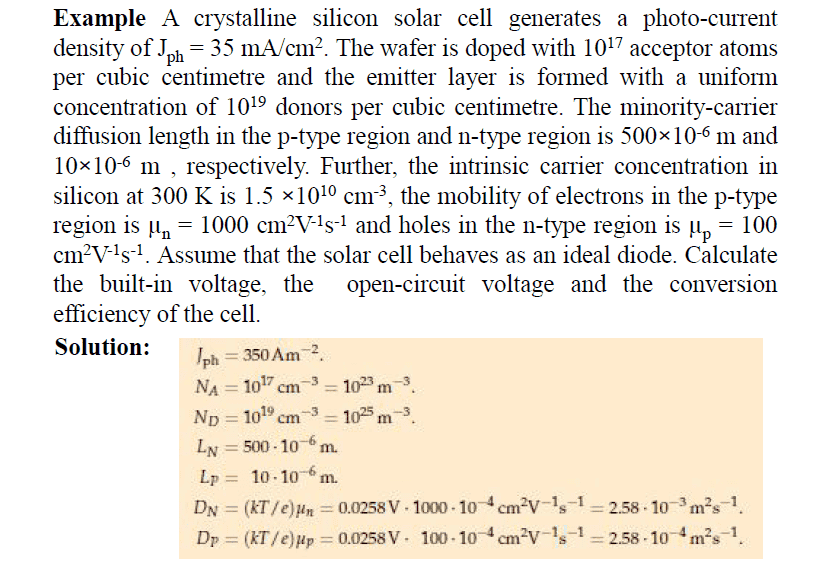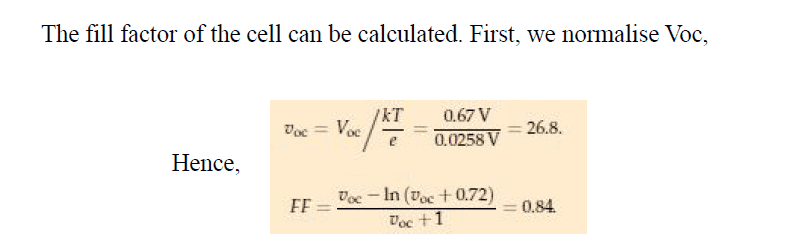# The fill factor of a solar cell

• patric44

#### patric44

Homework Statement
a question about the fill factor of a solar cell
Relevant Equations
FF = Vm*Im/Voc*Isc
hi guys
for a solar cell i know that the fill factor is given by the following relation :
$$FF = \frac{Vm*Im}{Voc*Isc}$$
but when solving the following example :the book used the following relation in the problem?! :where this Ln relation came from ?

any help on this , i just want a hint how to derive it